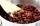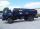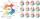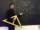# Mean + weighted average - math problems

#### Number of problems found: 26

• Dried fruitThe manufacturer produces a mixture of dried fruit. He purchased: 10kg pineapple for 200 Kc/kg 2kg papaya for 180 kc/kg 1kg of banana for 400 Kc/kg How many kgs of raisin for 80 Kc/kg must be put into the mix by the manufacturer so that the production pri
• Kerosine and petrolIf 4 liters of petrol containing 15% kerosine are added to another 7 liters of petrol containing 10% kerosine, what percentage of the petrol is kerosine?
• ContestantsIn the competition it was possible to get 0-5 points. In fact, 5 contestants of the 15 best contestants has earned 5 points or 4 points (which have won 10 contestants). The number of contestants who earned 3 points was the same as the number of contestant
• Center of gravityThe mass points are distributed in space as follows - specify by coordinates and weight. Find the center of gravity of the mass points system: A1 [1; -20; 3] m1 = 46 kg A2 [-20; 2; 9] m2 = 81 kg A3 [9
• CertificateThere is 31 students in a class. From mathematics was'nt worse mark than 2. Average mark in mathematics was 2. How many students have mark 1 and how many mark 2?
• WaterMix 67 l of water with a temperature of 67 °C, 17 l warm of 32 °C, and 79 l water of 87 °C. What is the temperature of the mixed water immediately after mixing?

We apologize, but in this category are not a lot of examples.
Do you have an exciting math question or word problem that you can't solve? Ask a question or post a math problem, and we can try to solve it.

We will send a solution to your e-mail address. Solved examples are also published here. Please enter the e-mail correctly and check whether you don't have a full mailbox.

Looking for help with calculating arithmetic mean? Mean - math problems. Weighted average - math problems.